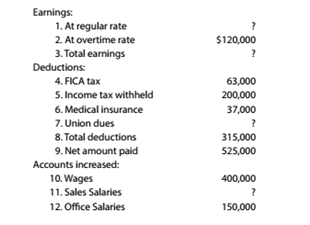Chapter 8, Problem 8.6E

Chapter
Section
Textbook Problem

Summary payroll data In the (blowing summary of data for a payroll period, some amounts have been intentionally omitted:Compute the amounts omitted in lines (I). (3). (7). and (II),

To determine

Concept Introduction:

Payroll means that amount of money that employer pays to the employees against the services provided by the employees to the employers. The payroll includes salaries and wages of the employees.

To compute:

The omitted amounts of the payroll data.

Explanation

The computations of omitted amounts are as follows:

Firstly, the total earnings are to be computed i.e. (3) :

Total Earnings=Total deductions+Net amount paid=$315,000+$525,000=$840,000 Now, the amount of earnings at regular rate is to be computed i.e. (1) : Earnings at Regular Rate=Total earningsEarnings at overtime rate=$840,000$120,000=$720,000

Now, the amount of union dues is to be calculated i.e. (7) :

Union Dues=Total deductions(FICA tax+Income tax withheld+

Still sussing out bartleby?

Check out a sample textbook solution.

See a sample solution

The Solution to Your Study Problems

Bartleby provides explanations to thousands of textbook problems written by our experts, many with advanced degrees!

Get Started

Briefly describe the difference between a job order cost system and a process cost system.

College Accounting, Chapters 1-27 (New in Accounting from Heintz and Parry)

Finally, you can also use the information on the Internet to value the entire corporation. This approach requir...

Fundamentals of Financial Management, Concise Edition (with Thomson ONE - Business School Edition, 1 term (6 months) Printed Access Card) (MindTap Course List)

What is a 10-K?

Cornerstones of Financial Accounting